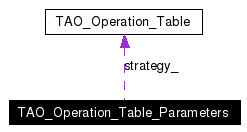# TAO_Operation_Table_Parameters Class Reference

Parameters used to create the operation table. More...

`#include <Operation_Table.h>`

Collaboration diagram for TAO_Operation_Table_Parameters:[legend]
List of all members.

## Public Types

enum  DEMUX_STRATEGY {
TAO_LINEAR_SEARCH, TAO_DYNAMIC_HASH, TAO_PERFECT_HASH, TAO_BINARY_SEARCH,
TAO_ACTIVE_DEMUX, TAO_USER_DEFINED
}
various lookup strategies More...

## Public Member Functions

void lookup_strategy (DEMUX_STRATEGY s)
Set the lookup strategy from the list of enumerated values.
DEMUX_STRATEGY lookup_strategy (void) const
void concrete_strategy (TAO_Operation_Table *ot)
TAO_Operation_Tableconcrete_strategy (void)
Return the operation table that is being used to do the lookup.
TAO_Operation_Table_Parameters (void)
Constructor.
~TAO_Operation_Table_Parameters (void)
Destructor.

## Private Attributes

TAO_Operation_Tablestrategy_
Pointer to the object that implements a lookup strategy.
DEMUX_STRATEGY type_
The enumerated value indicating the lookup strategy.

## Detailed Description

Parameters used to create the operation table.

## Member Enumeration Documentation

various lookup strategies

Enumeration values:
 TAO_LINEAR_SEARCH TAO_DYNAMIC_HASH TAO_PERFECT_HASH TAO_BINARY_SEARCH TAO_ACTIVE_DEMUX TAO_USER_DEFINED

## Constructor & Destructor Documentation

 TAO_Operation_Table_Parameters::TAO_Operation_Table_Parameters ( void )
 Constructor.

 TAO_Operation_Table_Parameters::~TAO_Operation_Table_Parameters ( void )
 Destructor.

## Member Function Documentation

 TAO_Operation_Table * TAO_Operation_Table_Parameters::concrete_strategy ( void )
 Return the operation table that is being used to do the lookup.

 void TAO_Operation_Table_Parameters::concrete_strategy ( TAO_Operation_Table * ot )
 Provide a data structure that will do the lookup. This is useful for user-defined lookup strategies.

 TAO_Operation_Table_Parameters::DEMUX_STRATEGY TAO_Operation_Table_Parameters::lookup_strategy ( void ) const
 Return the enumerated value for the lookup strategy. Default is Dynamic Hashing.

 void TAO_Operation_Table_Parameters::lookup_strategy ( DEMUX_STRATEGY s )
 Set the lookup strategy from the list of enumerated values.

## Member Data Documentation

 TAO_Operation_Table* TAO_Operation_Table_Parameters::strategy_` [private]`
 Pointer to the object that implements a lookup strategy.

 DEMUX_STRATEGY TAO_Operation_Table_Parameters::type_` [private]`
 The enumerated value indicating the lookup strategy.

The documentation for this class was generated from the following files:
Generated on Thu Feb 10 20:45:25 2005 for TAO_PortableServer by1.3.9.1